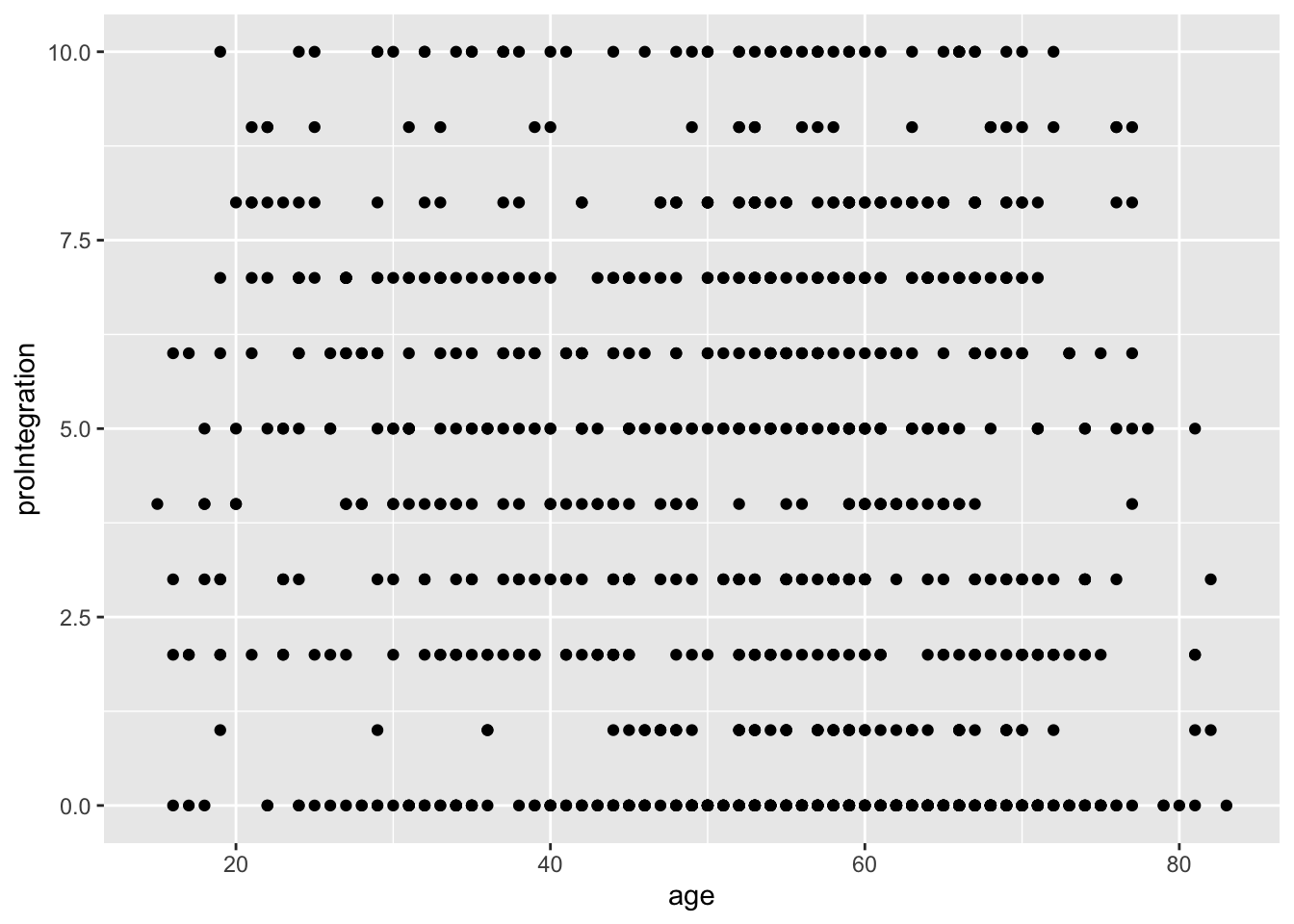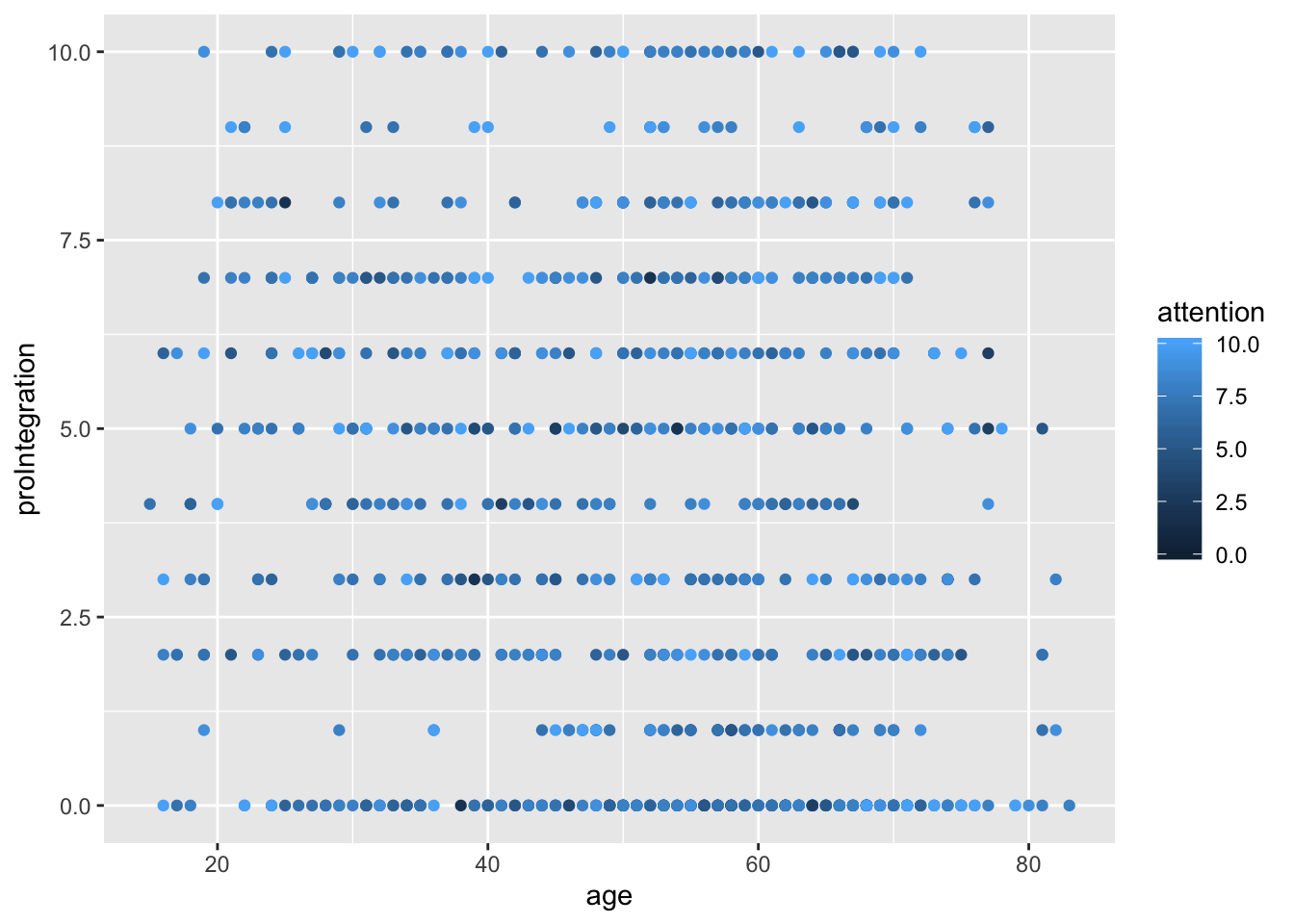1 Introduction

We continue with the same data set we used in Lab 1, which we can download directly from the web server using the “rio” package:

library(rio)
library(pander)
library(stargazer)
##
##  Hlavac, Marek (2018). stargazer: Well-Formatted Regression and Summary Statistics Tables.
##  R package version 5.2.2. https://CRAN.R-project.org/package=stargazer
library(dplyr)
##
## Attaching package: 'dplyr'
## The following objects are masked from 'package:stats':
##
##     filter, lag
## The following objects are masked from 'package:base':
##
##     intersect, setdiff, setequal, union
library(ggplot2)

brexit <- import("http://www.joselkink.net/files/data/brexit_subset.Rdata")

In the previous two labs we looked at bar charts and various plots and statistics to summarize individual variables. In this lab we will make the first moves towards linear regression, the core topic of the course. We start out by looking at visualisation, including the scatter plot and the regression line, and then look at the more numerical estimation of the linear regression.

2 Visualisation of relationships

2.1 Scatter plots

Scatter plots are plots to look at the relationship between two continuous or scale variables. This can help to answer questions such as: “Does economic development increase support for democracy among a population?”, “Do higher tax rates lead to lower levels of corruption?”, or “Are voters with anti-immigrant attitudes more likely to vote for populist parties?”

In the scatter plot, each point represents one observation, whereby you can read the score on the two variables by looking at the position of the point relative to the x- and y-axis. We use the geom_point() function to generate the scatter plot.

ggplot(brexit, aes(x = age, y = proIntegration)) + geom_point()We can now add features using the same aesthetic mapping, for example changing color by whether a respondent pays attention to politics or not.

ggplot(brexit) + geom_point(aes(x = age, y = proIntegration, color = attention))While we can use a categorical variable to change the color, shape, transparency, etc. of each of the points, we can also decide to make a raster of graphs, one for each category. For example below we produce the above plot for each party voter separately.

ggplot(brexit) +
geom_point(aes(x = age, y = proIntegration, color = attention)) +
facet_wrap(~ party)